1. Introduction

This document is an attempt to describe the design of the Alan IF Language System. At this point it is very limited and only describes a few aspects. It was created so that any sudden impulses to describe anything that concerns the design of Alan should have a natural place, hopefully making it more probable that such impulses actually resulted in something.

2. Overview

The Alan IF Language System consists of a compiler and an interpreter. The compiler analyses the input source code (following the Alan language specification) and transforms it to a format that is suitable as input to the interpreter. That format is referred to as the Acode.

The interpreter reads that Acode, constructs some internal structures from it and then starts “executing” it. In this case “executing” means interpreting specific sections of the Acode, mixing that with asking the player for input and analyzing that to see what other parts of the Acode needs to be interpreted.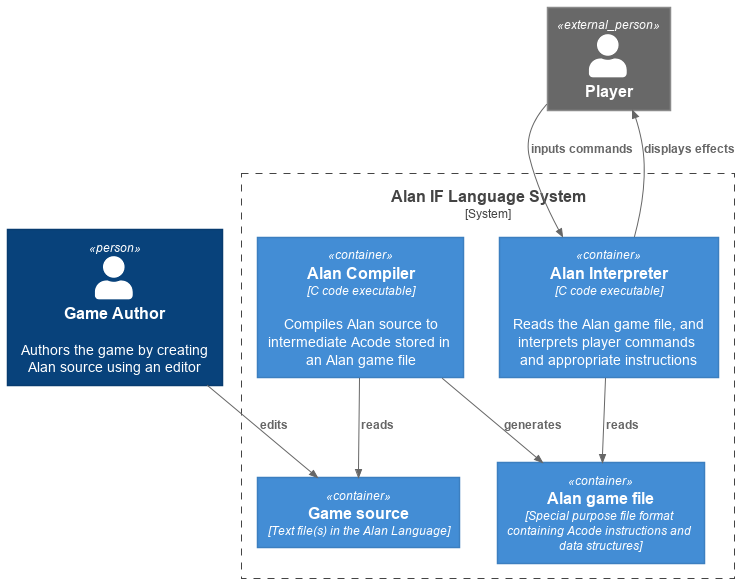2.1. The Compiler

The compiler is structured much like any other compiler. It has a scanner/lexer which turns text into symbols, a parser which ensures that input follows the grammar of the language, and as it does that it also builds an internal representation of the input, an Abstract Syntax Tree (AST). The AST is then traversed to do some semantic analysis, cross-referencing and “decorating”, and also building a symbol table of entities represented by the input. Finally that decorated AST it traversed and the output, in this case Acode, is generated.

2.2. The Interpreter

The interpreter, when started by the player, loads a file which is the result of a compilation by the Alan compiler. The file consists of:

• data structures for player command parsing, including all words that can be used

• data structures describing the “world”, locations, objects, etc.

• data strucrures for rules and events

• instructions to be executed in various circumstances, like the checks and bodies for verbs, events, etc.

The steps in an interpreter “round” is described in The Manual.

3. Language Design

3.2. Articles

Instances are mentioned and described in a number of situations. Sometimes it is necessary to refer to an instance in an indefinite form, and sometimes in a definite form. E.g.

I can’t open <indefinite form> with a spanner.
You can’t take <definite form>.

INDEFINITE & DEFINITE forms are required:

The article Isa b
Definite
Article "the".
Indefinite Article "an"
End The article.

The sak Isa x
Definite
Form "saken".
Indefinite
Article "en"

Not all languages have definite and indefinite articles that work well:

Definite "den"
Indefinite "en"
Mentioned "stol"

So we also allow

Name stol
Definite Form "stolen"
Indefinite Form "en stol"

The Mentioned clause should be in indefinite form. To say a Definite or Indefinite form of the instance:

1. If that Form is available it will be used, else

2. The Article followed by the Mentioned will be used

With corresponding Say statements:

 Say o. use Name ⇒ “stol” Say The o. use Definite ⇒ “stolen” Say A o. use Indefinite ⇒ “en stol” (Say Any o. use Plural ⇒ “stolar”??? Not needed, use "I can’t see any" Say o. "." for now.)

Since Mentioned is constructed from the first Name clause, the following would work:

Every person Isa actor
Definite Article ""
End Every person.

The mr_a Isa person
End The mr_a.

Problem is still what to say when:

I can’t see any mr andersson here.

But a library could:

Syntax talk_to = talk to (p)! Where p Isa person Else ...

Verb talk_to
Check p Is Here
Else "I can't see" Say The p. "here."
End Verb talk_to.

Verb talk_to

3.2.1. Singular and Plural

Do we really need all the four forms? Indefinite singular/plural and definite singular/plural?

3.2.2. The “Any” form

Possibly there is also a fifth form, as in “I can’t see any door here.” But let’s leave that for later. Possibly: Say Any x. & Say No x..

3.2.3. Pronouns

Sometimes a pronoun is possibly nice:

She does not want to talk to you.

However it is different in output and input. In input we would like to have “it” available for persons too, “her”. This can often be solved using synonyms.

The article Isa b
Definite
Article "the".
Pronoun "it".
Plural "articles"
Indefinite Form "any article"
...
The sak Isa x
Definite
Form "saken".
Pronoun "den".
Indefinite
Form "sak"
...

4. Detailed Design

4.2. The Interpreter

The interpreter starts by loading the game file into memory. It consists of two types of data, one being data structures of various kinds that the interpreter is inspecting, the other being sequences of “instructions” that the interpreter “executes”. The Acode (data structures and instructions) is structured in a fashion that closely supports the structure of the Alan Language.

This chapter describes those data structures and the instructions as well as particular algorithms that are used by the interpreter.

4.2.1. Common Data Structures

Since the compiler will generate data the interpreter will read, some data structures need to be known to both. The definitions of these are in interpreter/acode.h.

Parameter & Syntax Mapping Tables

Since multiple syntaxes may map to the same verb, a mapping between them is necessary. This mapping converts a syntax number into a verb code as well as remapping the parameter numbers into the “canonical” order for this verb.

The mapping is generated into two structures, the parameter order mapping, and the syntax-verb mapping table.

Parameter mapping

The parameter order mapping is simply a table with as many entries as there are parameters in the syntax, followed by an EOF. Each entry is an index into the “canonical” order of the parameters. As an example, the following is a parameter order mapping table for a syntax with three parameters in reverse canonical order and would occupy 4 consequtive Awords: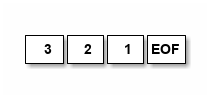This table is used to simply swap parameters so they carry the same meaning as in the “canonical” syntax.

Syntax-verb mapping

The syntax-verb mapping is a table of entries, one for each syntax. The correct syntax-verb mapping is found by matching the syntaxNumber found during command parsing with the one in each of the syntax-verb mapping entries. The entries have three fields, where parameterMapping points to a parameterMapping table as described above and the verbCode indicates which verb to execute.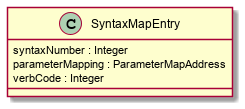The Dictionary

The dictionary holds all words the player is supposed to be able to input. A simple sequential lookup converts the string to an index into the dictionary array.

Each entry has the following general structure: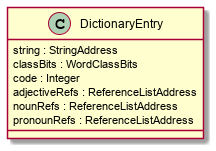4.2.3. Acode Instructions

The Acode instructions are designed around the model of a stack machine. A stack machine primarily uses a stack to manage its state and perform operations. E.g. an addition of two numbers will be performed by first “executing” two push operations to get the two numbers on the stack. Then the “add” operation is executed, which requires that there are two numbers already on the stack, which are replaces by the sum.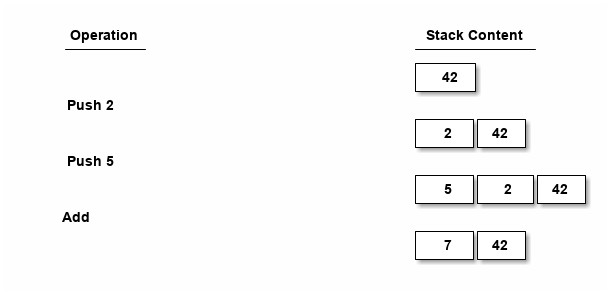As we can see each operation (“instruction”) is either removing something from the stack, and/or pushing something onto it.

Below is a list of instructions and details about their specifics.

LOOP

Use: start a loop

Context: on entry the stack contains from the top, starting loop value and the loop limit.

Function: Act as a loop start marker. When executed, check for loop termination, if so, go to end of the corresponding loop.

LOOP

Before

After

Loop index

Loop index

Upper limit of loop

Upper limit of loop

A loop value has to be calculated from the index since the index might be an index in a SET, such as when looping over the members in a set.

CALCULATE LOOP VALUE

Before

After

Loop value

Loop index

Loop index

Upper limit of loop

Upper limit of loop

Aggregate value

Aggregate value

LOOPNEXT

Context: none

Function: skip forward over instructions (possibly containing LOOP/LOOPEND-pairs) until next instruction to execute is an LOOPEND on the corresponding level.

LOOPNEXT

Before

After

Loop index

Loop index

Upper limit of loop

Upper limit of loop

LOOPEND

Use: test a loop for termination

Context: upper limit, and loop index on the top of the stack

Function: test the loop index against the upper limit. If not reached then increment index and back up until next instruction to execute is the first after the matching LOOP, else pop off the index and the limit and continue.

LOOPEND

Before

Continue

Terminate

Loop index

Incremented loop index

Upper limit of loop

Upper limit of loop

COUNT

Use: aggregate (using counting) number of items, usually instances, matching a set of filters

Context: at entry the three top values on the stack are the loop value, the loop index, and upper limit as the COUNT follows immediately on an AGRCHECK. Fourth value from the top is the aggregate value.

Function: Increment the fourth topmost value (the aggregate value).

COUNT

Before

After

Loop index

Loop index

Upper limit of loop

Upper limit of loop

Aggregate value

Updated aggregate value

MAX, MIN & SUM

Works exactly like COUNT, except at entry there is an extra value on the stack (at the top). This is the attribute value that the aggregation should use.

MAX, MIN & SUM

Before

After

Attribute value

Loop index

Loop index

Upper limit of loop

Upper limit of loop

Aggregate value

Updated aggregate value

4.3. Code Generation Principles

This chapter describes some language constructs that have more complicated mappings and for which some care need to be taken when generating the code, and possibly data, to represent and execute them.

4.3.1. Language Constructs

For Each

The For Each loop is generated into an initialization step, a filter step and the statements. A local variable is used since the loop-code might reference the loop value. Note that the loop value might be different from the loop index, e.g. when looping over a set of integers. The instructions used are:

• FRAME — start a new stack frame with one local variable

• LOOP — the loop start

• LOOPEND — test for and terminate loop

• ENDFRAME — drop stack frame with local variables

The code layout is as follows:

FRAME 1                               -- create a frame with 1 local
<calculate and push upper limit>
PUSH 1                                -- initial loop index
LOOP
<calculate loop value from index>
SETLOCAL 1                            -- save loop value in local var
GETLOCAL 1
<filter1>                             -- code to evaluate first filter
NOT                                   -- invert it
IF
LOOPNEXT                              -- if the filter did not match, next loop
ENDIF
GETLOCAL 1
<filter2>                             -- code to evaluate 2nd filter
NOT
IF
LOOPNEXT
ENDIF
...                                   -- and so on for each filter
LOOPEND                               -- loop test and termination
ENDFRAME
Aggregates

All aggregates are generated into a loop using the same structures as EACH. The instructions used are:

• LOOP — start loop

• LOOPNEXT — if filter inclusion was not true

• COUNT, MAX, MIN & SUM — actual aggregation performed

• LOOPEND — repeat or terminate aggregation loop

The code layout is as follows:

PUSH <maxint for MIN, 0 for all else> -- initial aggregate value
<calculate and push limit>
PUSH 1                                -- initial loop index
LOOP
<calculate loop value from index>
<filter1>                             -- code to evaluate first filter
NOT
IF
LOOPNEXT
ENDIF
<calculate loop value from index>
<filter2>
NOT
IF
LOOPNEXT
ENDIF
...
<get attribute to aggregate over>     -- for all except COUNT
<aggregate>                           -- COUNT, MIN, MAX or SUM
LOOPEND                               -- loop

Note that for this to work the aggregate instructions have to look deep in the stack to find the current aggregate value since this is stored at the bottom. On top is the usual looping data, i.e. the limit, the index and temporarily a loop value.